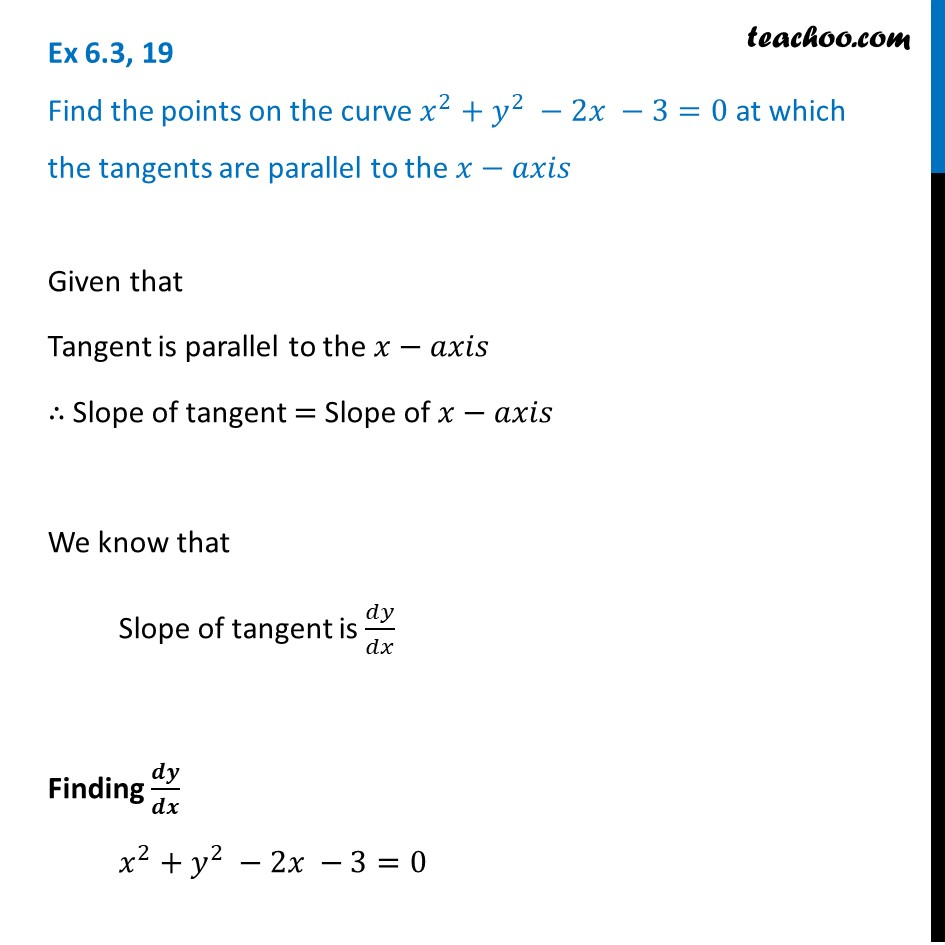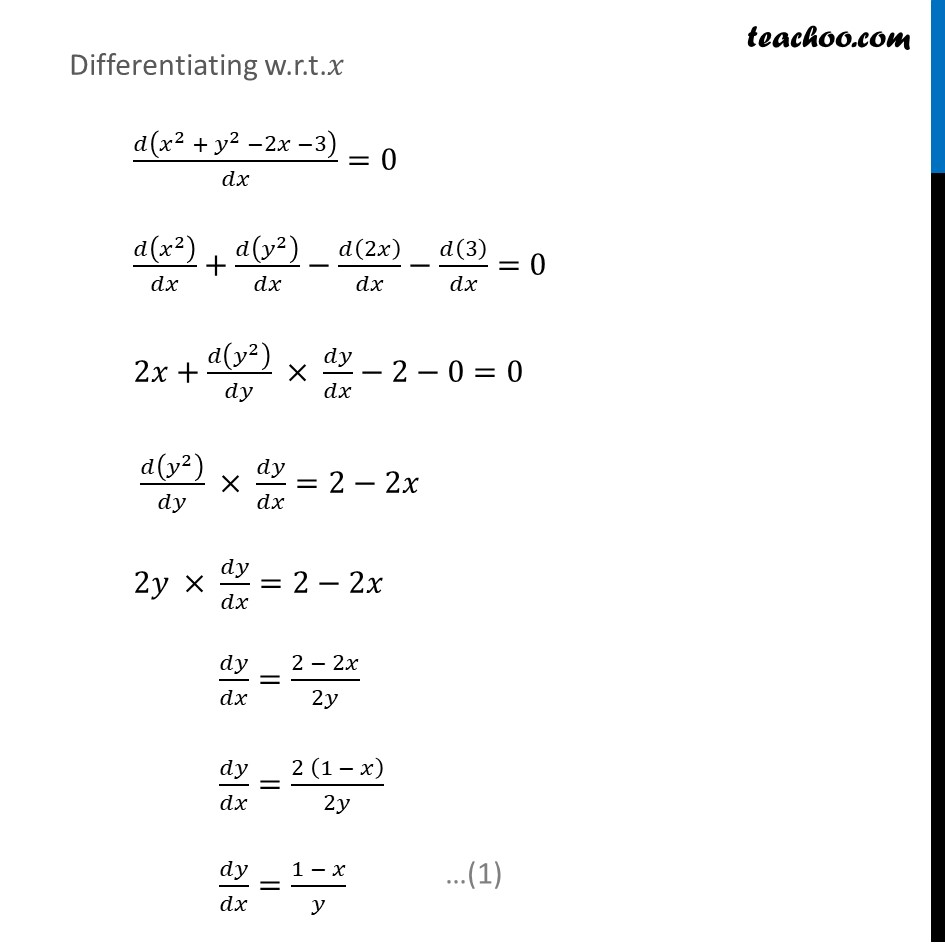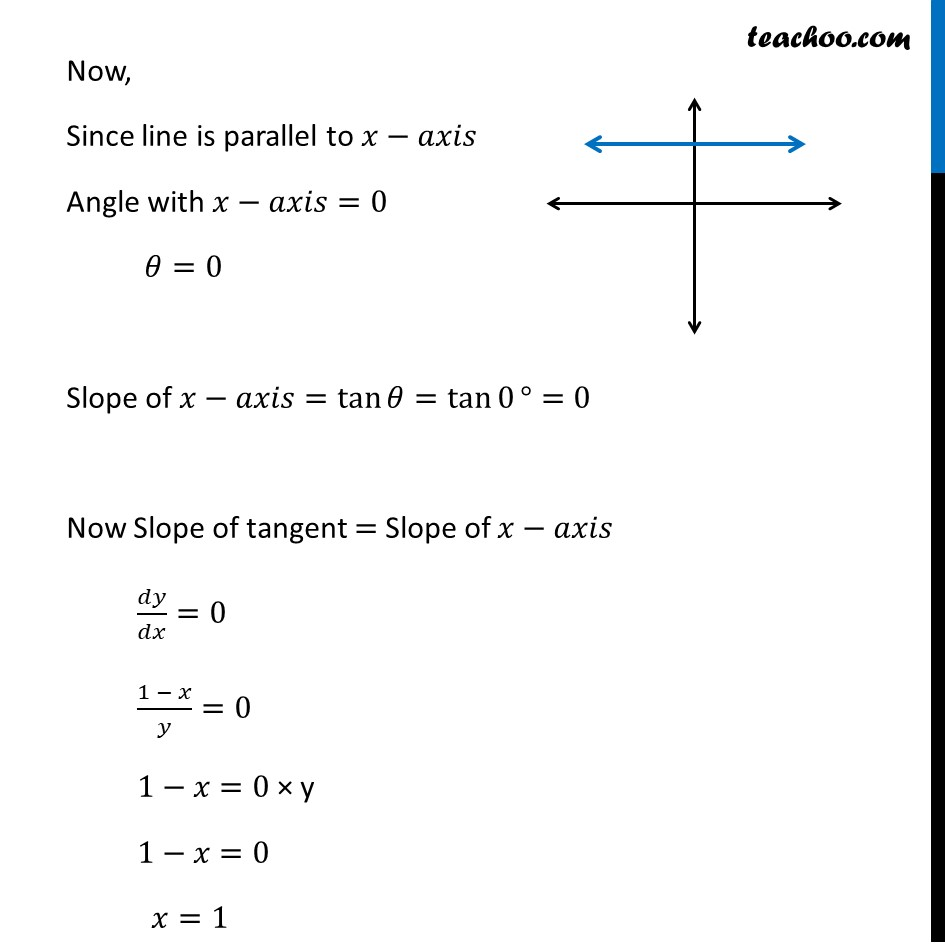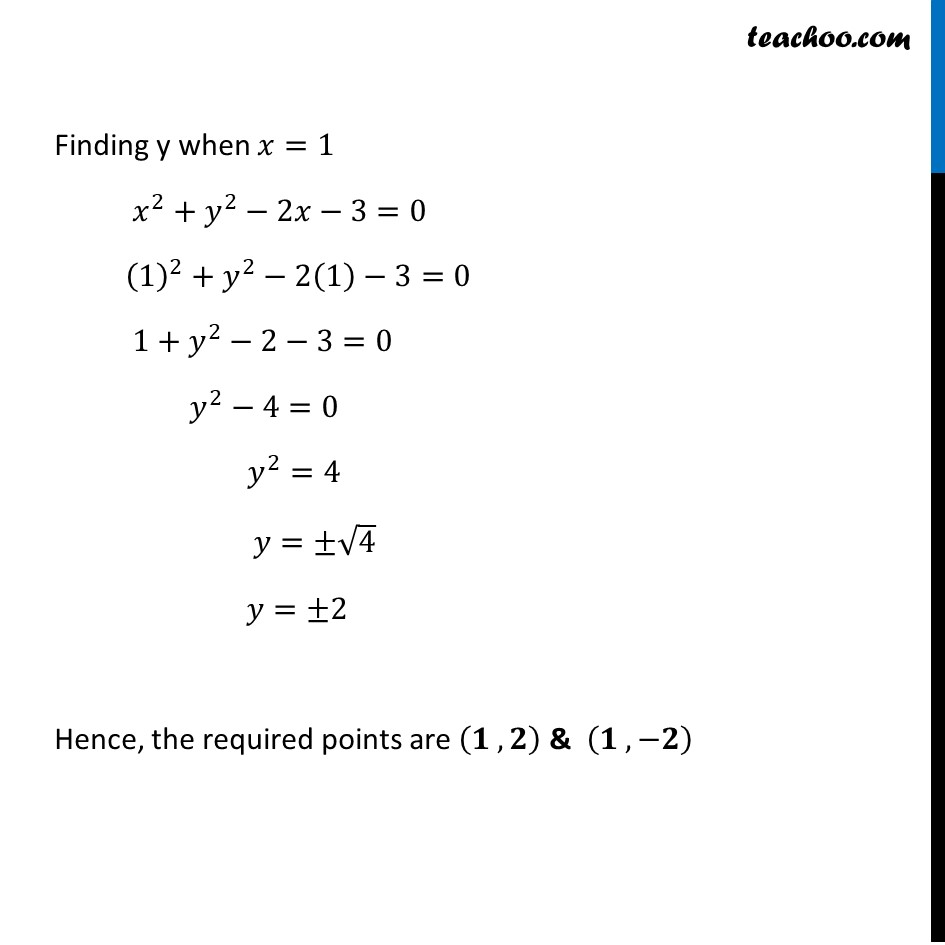Finding point when tangent is parallel/ perpendicular

Chapter 6 Class 12 Application of Derivatives
Concept wiseIntroducing your new favourite teacher - Teachoo Black, at only ₹83 per month

### Transcript

Ex 6.3, 19 Find the points on the curve 𝑥^2+𝑦^2 −2𝑥 −3=0 at which the tangents are parallel to the 𝑥−𝑎𝑥𝑖𝑠Given that Tangent is parallel to the 𝑥−𝑎𝑥𝑖𝑠 ∴ Slope of tangent = Slope of 𝑥−𝑎𝑥𝑖𝑠 We know that Slope of tangent is 𝑑𝑦/𝑑𝑥 Finding 𝒅𝒚/𝒅𝒙 𝑥^2+𝑦^2 −2𝑥 −3=0 Given that Tangent is parallel to the 𝑥−𝑎𝑥𝑖𝑠 ∴ Slope of tangent = Slope of 𝑥−𝑎𝑥𝑖𝑠 We know that Slope of tangent is 𝑑𝑦/𝑑𝑥 Finding 𝒅𝒚/𝒅𝒙 𝑥^2+𝑦^2 −2𝑥 −3=0 Differentiating w.r.t.𝑥 𝑑(𝑥^2 + 𝑦^2 −2𝑥 −3)/𝑑𝑥=0 𝑑(𝑥^2 )/𝑑𝑥+𝑑(𝑦^2 )/𝑑𝑥−𝑑(2𝑥)/𝑑𝑥−𝑑(3)/𝑑𝑥=0 2𝑥+𝑑(𝑦^2 )/𝑑𝑦 × 𝑑𝑦/𝑑𝑥−2−0=0 𝑑(𝑦^2 )/𝑑𝑦 × 𝑑𝑦/𝑑𝑥=2−2𝑥 2𝑦 × 𝑑𝑦/𝑑𝑥=2−2𝑥 𝑑𝑦/𝑑𝑥=(2 − 2𝑥)/2𝑦 𝑑𝑦/𝑑𝑥=(2 (1 − 𝑥))/2𝑦 𝑑𝑦/𝑑𝑥=(1 − 𝑥)/𝑦 Now, Since line is parallel to 𝑥−𝑎𝑥𝑖𝑠 Angle with 𝑥−𝑎𝑥𝑖𝑠=0 𝜃=0 Slope of 𝑥−𝑎𝑥𝑖𝑠=tan⁡𝜃=tan⁡0°=0 Now Slope of tangent = Slope of 𝑥−𝑎𝑥𝑖𝑠 𝑑𝑦/𝑑𝑥=0 (1 − 𝑥)/𝑦=0 1−𝑥=0 × y 1−𝑥=0 𝑥=1 Finding y when 𝑥=1 𝑥^2+𝑦^2−2𝑥−3=0 (1)^2+𝑦^2−2(1)−3=0 1+𝑦^2−2−3=0 𝑦^2−4=0 𝑦^2=4 𝑦=±√4 𝑦=±2 Hence, the required points are (𝟏 , 𝟐) & (𝟏 , −𝟐)